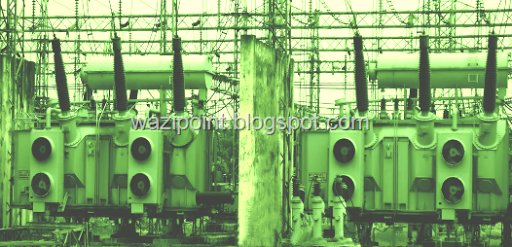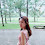WAZIPOINT Engineering Science & Technology: Transformer Capacity and Rating

## What Is The Difference Between Capacity And Rating Of Transformer ?

Here is three simple terms- Transformer, Capacity and Rating. Transformer is the targeted equipment where capacity is loading size and  rating denotes working ability.

Most of the Electrical Engineer are well known about transformer and it’s capacity or ratings; hope this article will helpful for those are not engineering background but dealing technical matters, like- one of my business associate asking a couple of weeks ago in a business talk, “what is transformer capacity and ratings?”.

##Before knowing the difference between capacity and ratings we would like to understand the basic definition of transformer. Simply a person or thing that transforms something from one place to others is a transformer. In Electrical Engineering field a transformer is defined as an electrical apparatus or equipment for transferring electrical energy from one circuit to others reducing or increasing voltage of an alternating current is known as Transformer.

In use of practice, transformer may various types depending of different factors like voltage step-up/down, energy transmission/distribution, transmission line phase, handling power or equipment, Cooling system of transformer etc..
Step-up Transformer/ Step-down Transformer;
• Single Phase / Three phase Transformer;
• Power transformer/ distribution transformer/ equipment transformer;
• Liquid cooling/ dry cooling; etc..

#### Uses and Application of Transformer

The most important uses and application of Transformer are:
1. It can rise or lower the level of level of Voltage or Current ( when voltage increases, current decreases and vice versa because P =V x I, and Power is same ) in an AC Circuit;
2. It can increase or decrease the value of capacitor, an inductor or resistance in an AC circuit. It can thus act as an impedance transferring device;
3. It can be used to prevent DC from passing from one circuit to the other;
4. it can isolate two circuits electrically.

## What Is Transformer Capacity?

Transformer capacity is as simple as others capacity means; shortly we could say transformer capacity denotes how much energy can flow/transfer safely.
The transformer capacity measuring unit is little bit different from other electrical equipment. It is not measured as Kilo-Watt (kW), it’s unit is Kilo-Volt-Ampere (kVA).
Typically transformer capacity is mentioned as MVA, KVA, or in basic VA, where V for voltage, A for Ampere, K for kilo and M for mega.
The reason for VA rather watt is that amperage can carry at a specific voltage, but a percentage of apparent power kVA is used to carry real power kW, the fact is calculated as Power Factor.
If you interested to Easy Understanding of Power Factor, could visit the other page title- Electrical Power Factor in Schematic Diagram
Power Factor actually reduce the real power and case of higher rating equipment, end-user loser to pay extra electric bill and even penalty due to poor power factor. Utility company increases user to improve power factor using some power factor improvement equipment like- PFI unit or capacitive load.

For industrial load to improve power factor promptly harmonic filter and power factor improvement unit is very important. To understand detail about Harmonic Filter you could read other article

### What Is Transformer Ratings?

Transformer Rating is denote the transformer voltage level. Some times it very much similar to capacity and ratings, but an important difference is there, transformer capacity may same in different rated  voltage.

Simple example- 10MVA transformer, rated voltage 132kV/33kV; means transformer load transfer capacity (Voltage X Current) is 10MVA and rated voltage primary feeding voltage is 132kilo Volt and output voltage is 33 kilo-Volt. Transformer, primary (Voltage X Current) = secondary (Voltage X Current)=10MVA.

similarly 10MVA transformer, rated voltage 33kV/11kV; means transformer load transfer capacity (Voltage X Current) is 10MVA and rated voltage primary feeding voltage is 33kilo Volt and output voltage is 11 kilo-Volt. Transformer, primary (Voltage X Current) = secondary (Voltage X Current)=10MVA.

So, above both transformer capacity is same, but ratings are different.
The interesting information is that-
Electrical  transformer is a static device which transforms electrical energy from one circuit to another circuit without any direct connection.  The magic is happen with the help of mutual induction between two winding”.

Hope this makes sense the basic definition of electrical apparatus transformer and difference between  capacity and ratings of transformer.

#### 1 comment:

1.WAZIPOINT:
Thank you very much to visit and valuable comments on this blog post. Keep in touch for next and new article. Share your friends and well-wisher, share your idea to worldwide.